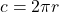## Tommy runs around a track whose circumference is 400 meters. He runs a single lap in a time of 62 seconds. What is Tommy’s displacement

Question

Tommy runs around a track whose circumference is 400 meters. He runs a single lap in a time of 62 seconds. What is Tommy’s displacement

in progress 0
2 months 2021-07-24T04:54:35+00:00 1 Answers 0 views 0

Explanation:

for circular motion we are expected to solve for Angular Displacement it is measured in radian

Measurement of Angular Displacement.

we can measure it using the following relation

∅= s/r

where

s = the distance travelled by the body, and

r = radius of the circle along which it is moving.

given that

circumference c,  s= 400 m

r= ?

we have to solve for the radius

we know that circumference400= 2*3.142*r

400= 6.282*r

divide both sides by 6.284 we have

400/6.284

r= 63.63 m

Angular  displcament

∅= 400/63.63